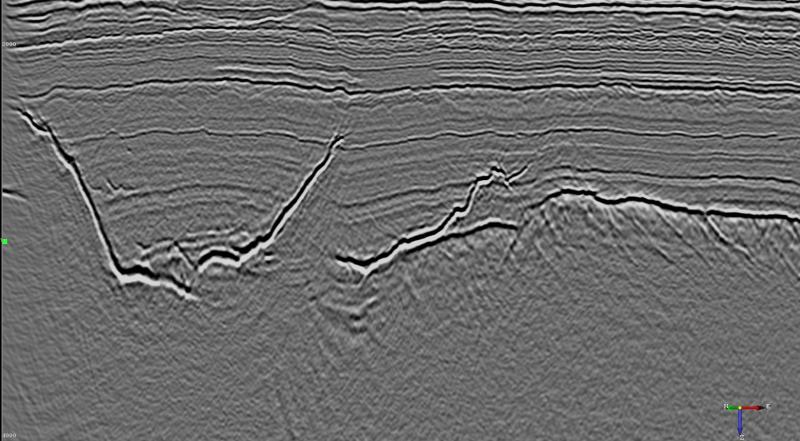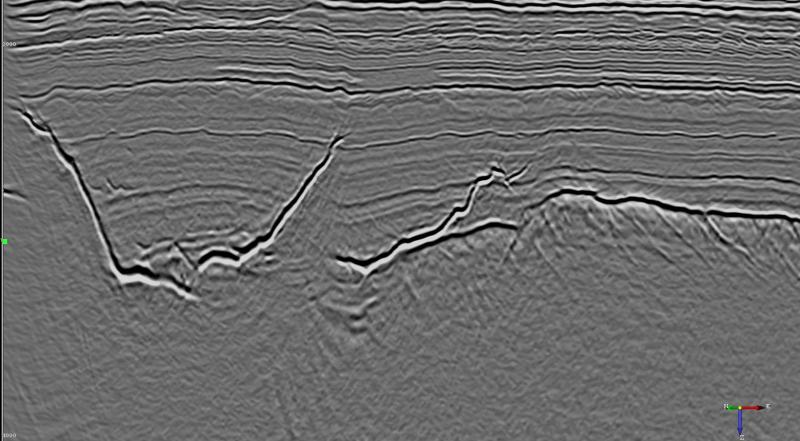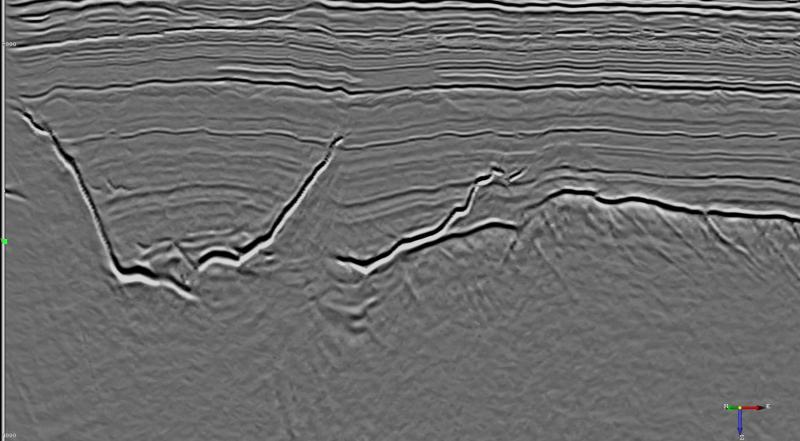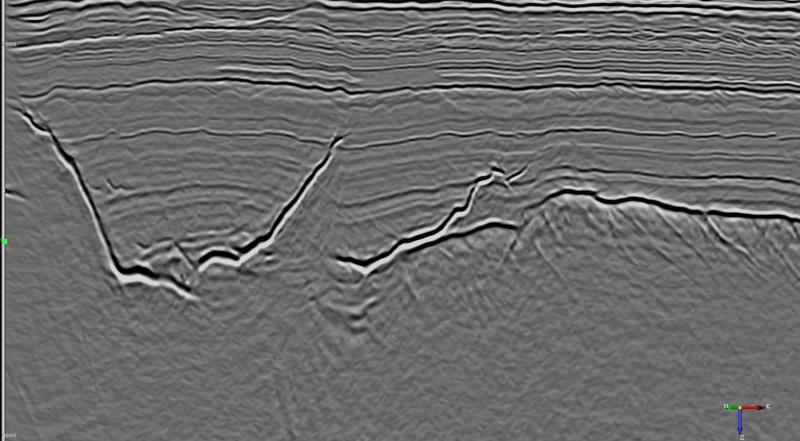This attribute plugin for the open source seismic interpretation platform OpendTect applies a structure preserving Mean of Least Variance filter.

### Description

This attribute is an implementation of a mean of least variance filter Schulze & Pearce (1993) where the analysis elements are all the possible planes through the sample points in the analysis block. This algorithm may be similar to that proposed by Al-Dossary & Wang (2011).

The sample variance for all samples on each analysis element is calculated and the output statistic (average, mean or element index) is output for the element with the least variance.

The following figure shows the relationship between the geometry of the analysis elements and the element index.

### Examples